Algebra

Equations

Inequalities

Graphs

Numbers

Calculus

Matrices

Tutorials

#### Algebra

The algebra section of QuickMath allows you to manipulate mathematical expressions in all sorts of useful ways. At the moment, QuickMath can expand, factor or simplify virtually any expression, cancel common factors within fractions, split fractions up into smaller ('partial') fractions and join two or more fractions together into a single fraction. More specialized commands are on the way.

#### What is algebra?

The term 'algebra' is used for many things in mathematics, but in this section we'll just be talking about the sort of algebra you come across at high-school.

Algebra is the branch of elementary mathematics which uses symbols to stand for unknown quantities. In a more basic sense, it consists of solving equations or manipulating expressions which contain symbols (usually letters, like x, y or z) as well as numbers and functions. Although solving equations is really a part of algebra, it is such a big area that it has its own section in QuickMath.

This part of QuickMath deals only with algebraic expressions. These are mathematical statements which contain letters, numbers and functions, but no equals signs. Here are a few examples of simple algebraic expressions :

x2-1

x2-2x+1

ab2+3a3b-5ab

x3+1

 1a + b + 1a - b
 x2-1x + 1

#### Expand

The expand command is used mainly to rewrite polynomials with all brackets and whole number powers multiplied out and all like terms collected together. In the advanced section, you also have the option of expanding trigonometric functions, expanding modulo any integer and leaving certain parts of the expression untouched whilst expanding the rest.

#### Factor

The factor command will try to rewrite an expression as a product of smaller expressions. It takes care of such things as taking out common factors, factoring by pairs, quadratic trinomials, differences of two squares, sums and differences of two cubes, and a whole lot more. The advanced section includes options for factoring trigonometric functions, factoring modulo any integer, factoring over the field of Gaussian integers (just the thing for those tricky sums of squares), and even extending the field over which factoring occurs with your own custom extensions.

Go to the Factor page

#### Simplify

Simplifying is perhaps the most difficult of all the commands to describe. The way simplification is performed in QuickMath involves looking at many different combinations of transformations of an expression and choosing the one which has the smallest number of parts. Amongst other things, the Simplify command will take care of canceling common factors from the top and bottom of a fraction and collecting like terms. The advanced options allow you to simplify trigonometric functions or to instruct QuickMath to try harder to find a simplified expression.

Go to the Simplify page

#### Cancel

The cancel command allows you to cancel out common factors in the denominator and numerator of any fraction appearing in an expression. This command works by canceling the greatest common divisor of the denominator and numerator.

Go to the Cancel page

#### Partial Fractions

The partial fractions command allows you to split a rational function into a sum or difference of fractions. A rational function is simply a quotient of two polynomials. Any rational function can be written as a sum of fractions, where the denominators of the fractions are powers of the factors of the denominator of the original expression. This command is especially useful if you need to integrate a rational function. By splitting it into partial fractions first, the integration can often be made much simpler.

Go to the Partial Fractions page

#### Join Fractions

The join fractions command essentially does the reverse of the partial fractions command. It will rewrite a number of fractions which are added or subtracted as a single fraction. The denominator of this single fraction will usually be the lowest common multiple of the denominators of all the fractions being added or subtracted. Any common factors in the numerator and denominator of the answer will automatically be cancelled out.

# Introduction to Algebraic Functions

The notion of correspondence is encountered frequently in everyday life. For example, to each book in a library there corresponds the number of pages in the book. As another example, to each human being there corresponds a birth date. To cite a third example, if the temperature of the air is recorded throughout a day, then at each instant of time there is a corresponding temperature.

The examples of correspondences we have given involve two sets X and Y. In our first example, X denotes the set of books in a library and Y the set of positive integers. For each book x in X there corresponds a positive integer y, namely the number of pages in the book. In the second example, if we let X denote the set of all human beings and Y the set of all possible dates, then to each person x in X there corresponds a birth date y.

We sometimes represent correspondences by diagrams of the type shown in Figure 1.17, where the sets X and Y are represented by points within regions in a plane. The curved arrow indicates that the element y of Y corresponds to the element x of X. We have pictured X and Y as different sets. However, X and Y may have elements in common. As a matter of fact, we often have X = Y.

Our examples indicate that to each x in X there corresponds one and only one y in Y; that is, y is unique for a given x. However, the same element of Y may correspond to different elements of X. For example, two different books may have the same number of pages, two different people may have the same birthday, and so on.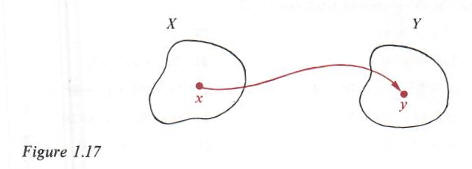In much of our work X and Y will be sets of real numbers. To illustrate, let X and Y both denote the set R of real numbers, and to each real number x let us assign its square x2. Thus to 3 we assign 9, to - 5 we assign 25, and so on. This gives us a correspondence from R to R. All the examples of correspondences we have given are functions, as defined below.

Definition

A function f from a set X to a set Y is a correspondence that assigns to each element x of X a unique element y of Y. The element y is called the image of x under f and is denoted by f(x). The set X is called the domain of the function. The range of the function consists of all images of elements of X.

Earlier, we introduced the notation f(x) for the element of Y which corresponds to x. This is usually read "f of x." We also call f(x) the value of f at x. In terms of the pictorial representation given earlier, we may now sketch a diagram as in Figure 1.18. The curved arrows indicate that the elements f(x), f(w), f(z), and f(a) of Y correspond to the elements x, y, z and a of X. Let us repeat the important fact that to each x in X there is assigned precisely one image f(x) in Y; however, different elements of X such as w and z in Figure 1.18 may have the same image in Y.

Beginning students are sometimes confused by the symbols f and f(x). Remember that f is used to represent the function. It is neither in X nor in Y. However, f(x) is an element of Y, namely the element which f assigns to x. Two functions f and g from X to Y are said to be equal, written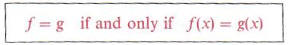for every x in X.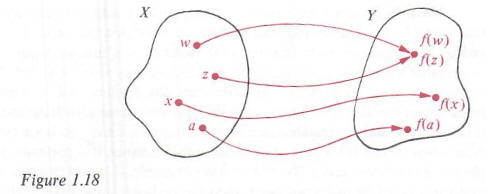Example 1 Let f be the function with domain R such that f(x) = x2 for every x in R. Find f(-6) and f(a), where a is any real number. What is the range of f?

Solution Values of f(or images under f) may be found by substituting for x in the equation  f(x) = x2 . Thus:If T denotes the range off, then by previous definition T consists of all numbers of the form f(a) where a is in R. Hence T is the set of all squares a2, where a is a real number. Since the square of any real number is nonnegative. T is contained in the set of all nonnegative real numbers. Moreover, every nonnegative real number c is an image underf, since. Hence the range of f is the set of all nonnegative real numbers.

If a function is defined as in the preceding example, the symbol used for the variable is immaterial; that is, expressions such as: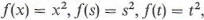and so on, all define the same function. This is true because if a is any number in the domain of f, then the same image a2 is obtained no matter which expression is employed.

Example 2 Let X denote the set of nonnegative real numbers and let f be the function from X to R defined by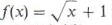for every x in X. Find f(4) and f(pi). If b and c are in X, find f(b + c) and f(b) + f(c).

Solution As in Example 1, finding images under f is simply a matter of substituting the appropriate number for x in the expression for f(x). Thus: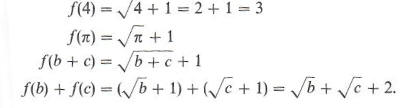Many formulas which occur in mathematics and the sciences determine functions. As an illustration, the formula A = pi*r2 for the area A of a circle of radius r assigns to each positive real number r a unique value of A. This determines a function f, where f(r) = pi*r2, and we may write A= f(r). The letter r, which represents an arbitrary number from the domain off, is often called an independent variable. The letter A, which represents a number from the range off, is called a dependent variable, since its value depends on the number assigned tor. When two variables r and A are related in this manner, it is customary to use the phrase A is a function of r. To cite another example, if an automobile travels at a uniform rate of 50 miles per hour, then the distance d (miles) traveled in time t (hours) is given by d = 50t and hence the distance d is a function of time t.

We have seen that different elements in the domain of a function may have the same image. If images are always different, then, as in the next definition, the function is called one-to-one.